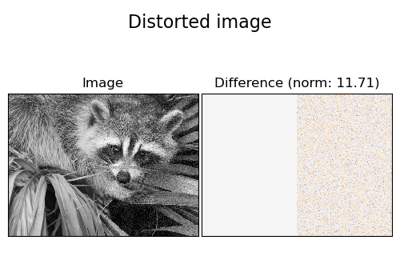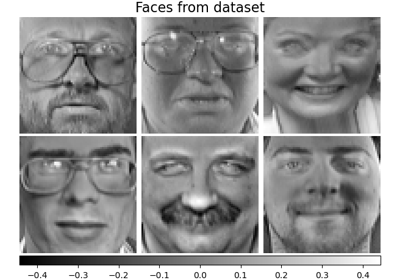# sklearn.decomposition.MiniBatchDictionaryLearning¶

class sklearn.decomposition.MiniBatchDictionaryLearning(n_components=None, *, alpha=1, n_iter=1000, fit_algorithm='lars', n_jobs=None, batch_size=3, shuffle=True, dict_init=None, transform_algorithm='omp', transform_n_nonzero_coefs=None, transform_alpha=None, verbose=False, split_sign=False, random_state=None, positive_code=False, positive_dict=False, transform_max_iter=1000)[source]

Mini-batch dictionary learning

Finds a dictionary (a set of atoms) that can best be used to represent data using a sparse code.

Solves the optimization problem:

(U^*,V^*) = argmin 0.5 || X - U V ||_2^2 + alpha * || U ||_1
(U,V)
with || V_k ||_2 = 1 for all  0 <= k < n_components


Read more in the User Guide.

Parameters
n_componentsint, default=None

Number of dictionary elements to extract.

alphafloat, default=1

Sparsity controlling parameter.

n_iterint, default=1000

Total number of iterations to perform.

fit_algorithm{‘lars’, ‘cd’}, default=’lars’

The algorithm used:

• 'lars': uses the least angle regression method to solve the lasso problem (linear_model.lars_path)

• 'cd': uses the coordinate descent method to compute the Lasso solution (linear_model.Lasso). Lars will be faster if the estimated components are sparse.

n_jobsint, default=None

Number of parallel jobs to run. None means 1 unless in a joblib.parallel_backend context. -1 means using all processors. See Glossary for more details.

batch_sizeint, default=3

Number of samples in each mini-batch.

shufflebool, default=True

Whether to shuffle the samples before forming batches.

dict_initndarray of shape (n_components, n_features), default=None

initial value of the dictionary for warm restart scenarios

transform_algorithm{‘lasso_lars’, ‘lasso_cd’, ‘lars’, ‘omp’, ‘threshold’}, default=’omp’

Algorithm used to transform the data:

• 'lars': uses the least angle regression method (linear_model.lars_path);

• 'lasso_lars': uses Lars to compute the Lasso solution.

• 'lasso_cd': uses the coordinate descent method to compute the Lasso solution (linear_model.Lasso). 'lasso_lars' will be faster if the estimated components are sparse.

• 'omp': uses orthogonal matching pursuit to estimate the sparse solution.

• 'threshold': squashes to zero all coefficients less than alpha from the projection dictionary * X'.

transform_n_nonzero_coefsint, default=None

Number of nonzero coefficients to target in each column of the solution. This is only used by algorithm='lars' and algorithm='omp' and is overridden by alpha in the omp case. If None, then transform_n_nonzero_coefs=int(n_features / 10).

transform_alphafloat, default=None

If algorithm='lasso_lars' or algorithm='lasso_cd', alpha is the penalty applied to the L1 norm. If algorithm='threshold', alpha is the absolute value of the threshold below which coefficients will be squashed to zero. If algorithm='omp', alpha is the tolerance parameter: the value of the reconstruction error targeted. In this case, it overrides n_nonzero_coefs. If None, default to 1.

verbosebool, default=False

To control the verbosity of the procedure.

split_signbool, default=False

Whether to split the sparse feature vector into the concatenation of its negative part and its positive part. This can improve the performance of downstream classifiers.

random_stateint, RandomState instance or None, default=None

Used for initializing the dictionary when dict_init is not specified, randomly shuffling the data when shuffle is set to True, and updating the dictionary. Pass an int for reproducible results across multiple function calls. See Glossary.

positive_codebool, default=False

Whether to enforce positivity when finding the code.

New in version 0.20.

positive_dictbool, default=False

Whether to enforce positivity when finding the dictionary.

New in version 0.20.

transform_max_iterint, default=1000

Maximum number of iterations to perform if algorithm='lasso_cd' or 'lasso_lars'.

New in version 0.22.

Attributes
components_ndarray of shape (n_components, n_features)

Components extracted from the data.

inner_stats_tuple of (A, B) ndarrays

Internal sufficient statistics that are kept by the algorithm. Keeping them is useful in online settings, to avoid losing the history of the evolution, but they shouldn’t have any use for the end user. A (n_components, n_components) is the dictionary covariance matrix. B (n_features, n_components) is the data approximation matrix.

n_iter_int

Number of iterations run.

iter_offset_int

The number of iteration on data batches that has been performed before.

random_state_RandomState instance

RandomState instance that is generated either from a seed, the random number generattor or by np.random.

Notes

References:

J. Mairal, F. Bach, J. Ponce, G. Sapiro, 2009: Online dictionary learning for sparse coding (https://www.di.ens.fr/sierra/pdfs/icml09.pdf)

Examples

>>> import numpy as np
>>> from sklearn.datasets import make_sparse_coded_signal
>>> from sklearn.decomposition import MiniBatchDictionaryLearning
>>> X, dictionary, code = make_sparse_coded_signal(
...     n_samples=100, n_components=15, n_features=20, n_nonzero_coefs=10,
...     random_state=42)
>>> dict_learner = MiniBatchDictionaryLearning(
...     n_components=15, transform_algorithm='lasso_lars', random_state=42,
... )
>>> X_transformed = dict_learner.fit_transform(X)


We can check the level of sparsity of X_transformed:

>>> np.mean(X_transformed == 0)
0.87...


We can compare the average squared euclidean norm of the reconstruction error of the sparse coded signal relative to the squared euclidean norm of the original signal:

>>> X_hat = X_transformed @ dict_learner.components_
>>> np.mean(np.sum((X_hat - X) ** 2, axis=1) / np.sum(X ** 2, axis=1))
0.10...


Methods

 fit(X[, y]) Fit the model from data in X. fit_transform(X[, y]) Fit to data, then transform it. get_params([deep]) Get parameters for this estimator. partial_fit(X[, y, iter_offset]) Updates the model using the data in X as a mini-batch. set_params(**params) Set the parameters of this estimator. Encode the data as a sparse combination of the dictionary atoms.
fit(X, y=None)[source]

Fit the model from data in X.

Parameters
Xarray-like of shape (n_samples, n_features)

Training vector, where n_samples in the number of samples and n_features is the number of features.

yIgnored
Returns
selfobject

Returns the instance itself.

fit_transform(X, y=None, **fit_params)[source]

Fit to data, then transform it.

Fits transformer to X and y with optional parameters fit_params and returns a transformed version of X.

Parameters
Xarray-like of shape (n_samples, n_features)

Input samples.

yarray-like of shape (n_samples,) or (n_samples, n_outputs), default=None

Target values (None for unsupervised transformations).

**fit_paramsdict

Additional fit parameters.

Returns
X_newndarray array of shape (n_samples, n_features_new)

Transformed array.

get_params(deep=True)[source]

Get parameters for this estimator.

Parameters
deepbool, default=True

If True, will return the parameters for this estimator and contained subobjects that are estimators.

Returns
paramsdict

Parameter names mapped to their values.

partial_fit(X, y=None, iter_offset=None)[source]

Updates the model using the data in X as a mini-batch.

Parameters
Xarray-like of shape (n_samples, n_features)

Training vector, where n_samples in the number of samples and n_features is the number of features.

yIgnored
iter_offsetint, default=None

The number of iteration on data batches that has been performed before this call to partial_fit. This is optional: if no number is passed, the memory of the object is used.

Returns
selfobject

Returns the instance itself.

set_params(**params)[source]

Set the parameters of this estimator.

The method works on simple estimators as well as on nested objects (such as Pipeline). The latter have parameters of the form <component>__<parameter> so that it’s possible to update each component of a nested object.

Parameters
**paramsdict

Estimator parameters.

Returns
selfestimator instance

Estimator instance.

transform(X)[source]

Encode the data as a sparse combination of the dictionary atoms.

Coding method is determined by the object parameter transform_algorithm.

Parameters
Xndarray of shape (n_samples, n_features)

Test data to be transformed, must have the same number of features as the data used to train the model.

Returns
X_newndarray of shape (n_samples, n_components)

Transformed data.

## Examples using sklearn.decomposition.MiniBatchDictionaryLearning¶Image denoising using dictionary learning¶Faces dataset decompositions¶